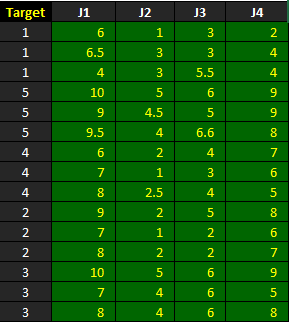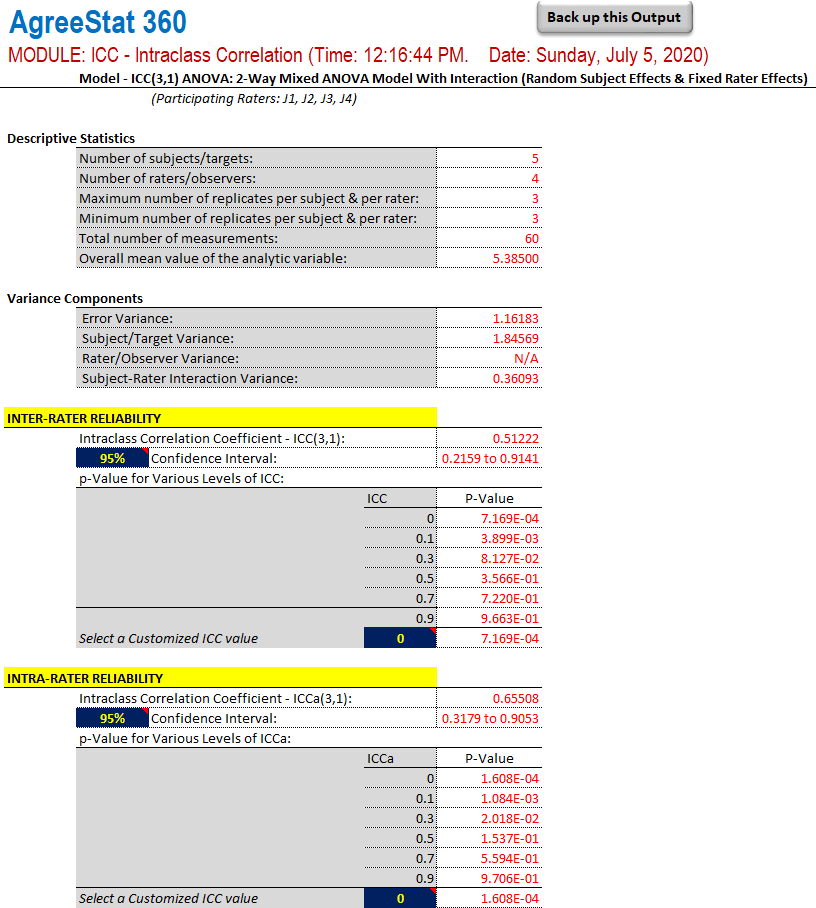﻿ AgreeStat/360: intraclass correlation coefficients for 3 raters or more under the two-way mixed effects modelAgreeStat Analytics

## Intraclass Correlation Coefficients for 3 Raters or More 2-Way Mixed Effects Model

Input Data

The dataset shown below is included in one of the example worksheets of AgreeStat 360 and can therefore be downloaded from the program (This video shows how you may import your own data to AgreeStat360). It contains the ratings that 4 judges J1, J2, J3, and J4 assigned to 5 subjects.

The objective is to use AgreeStat360 to compute intraclass correlation coefficients and associated precision measures under the 2-way mixed effects model.Analysis with AgreeStat/360

To see how AgreeStat360 processes this dataset to produce the intraclass correlation coefficients and associated precision measures, please play the video below.  This video can also be watched on youtube.com for more clarity if needed.

Results

The output that AgreeStat360 produces is shown below and has 4 parts. The basic descriptive statistics constitute the first part.  The second part shows different variance components, while the third and fourth parts shows the inter-rater and intra-rater reliability estimates respectively, along with their precision measures.

• Descriptive statistics: The first part of this output displays basic statistics about the number of non-missing observations for each variable as well as their mean values or number subjects.

• Variance components: This section will typically show the error variance, the subject variance, the rater variance, as well as the subject-rater interaction variance. These variance components are used for calculating the inter-rater and intra-rater reliability coefficients, and could help explain the magnitude of the reliability coefficients.

• Inter-rater reliability: This part shows the inter-rater reliability coefficient, its confidence interval and associated p-values. You can modify both the confidence level and the ICC null value to obtain the corresponding confidence intervals and p-values.  The blue cells contain dropdown lists contains new confidence levels and ICC null values to choose from.

• Intra-rater reliability
This part shows the intra-rater reliability coefficient, its confidence intervals and associated p-values.  Again, you can modify both the confidence level and the ICC null value to update the corresponding confidence intervals and p-values.